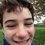# No S(n)>S(n^5)?

Inspired by this and this, I tried the same thing with n^5 and couldn't find an x that worked (or it is much much larger). Is there an x such that the sum of the digits of x is greater than the sum of the digits of x^5?Note by David Holcer
6 years, 4 months ago

This discussion board is a place to discuss our Daily Challenges and the math and science related to those challenges. Explanations are more than just a solution — they should explain the steps and thinking strategies that you used to obtain the solution. Comments should further the discussion of math and science.

When posting on Brilliant:

• Use the emojis to react to an explanation, whether you're congratulating a job well done , or just really confused .
• Ask specific questions about the challenge or the steps in somebody's explanation. Well-posed questions can add a lot to the discussion, but posting "I don't understand!" doesn't help anyone.
• Try to contribute something new to the discussion, whether it is an extension, generalization or other idea related to the challenge.

MarkdownAppears as
*italics* or _italics_ italics
**bold** or __bold__ bold
- bulleted- list
• bulleted
• list
1. numbered2. list
1. numbered
2. list
Note: you must add a full line of space before and after lists for them to show up correctly
paragraph 1paragraph 2

paragraph 1

paragraph 2

[example link](https://brilliant.org)example link
> This is a quote
This is a quote
    # I indented these lines
# 4 spaces, and now they show
# up as a code block.

print "hello world"
# I indented these lines
# 4 spaces, and now they show
# up as a code block.

print "hello world"
MathAppears as
Remember to wrap math in $$ ... $$ or $ ... $ to ensure proper formatting.
2 \times 3 $2 \times 3$
2^{34} $2^{34}$
a_{i-1} $a_{i-1}$
\frac{2}{3} $\frac{2}{3}$
\sqrt{2} $\sqrt{2}$
\sum_{i=1}^3 $\sum_{i=1}^3$
\sin \theta $\sin \theta$
\boxed{123} $\boxed{123}$

Sort by:

No output in 4 hours!! :(

- 6 years, 4 months ago

I crashed my computer doing this ... Nice question by the way

- 6 years, 4 months ago

Staff - 6 years, 4 months ago

Well I tried the same thing with S(n)>S(n^4) and it didn't work either.

- 6 years, 4 months ago

124499 works for the forth power. 1+2+4+4+9+9=29 124499**4=240250031031001002001. 2+4+2+5+3+1+3+1+1+2+1=25

- 6 years, 4 months ago

Oh It seems my computer can't handle such large numbers.

- 6 years, 4 months ago

Depends on programming language you are working upon

- 6 years, 4 months ago

Do you think there is a way to prove whether or not there exists an x for x^5?

- 6 years, 4 months ago

Probably there exists some large $x$ for which the inequality is satisfied. Probably finite solutions exist only when you consider $x^{k}$ for very very large $k$.

- 6 years, 4 months ago

Clearly if there is one such $x$ then there are infinitely many such $x$; just append zeroes at the end.

- 6 years, 4 months ago

Yes and this proves that either there are no solutions or there are infinite solutions.

- 6 years, 4 months ago

Yeah, but I mean one is not a power of ten times of the other. Otherwise, it would be pretty boring, wouldn't it?

- 6 years, 4 months ago

Not till now

- 6 years, 4 months ago

What, what number?!!

- 6 years, 4 months ago

I mean I don't know if there is any way to prove whether such n exists or not

- 6 years, 4 months ago

Oh, ok.Thnx.

- 6 years, 4 months ago